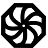# Programming-Idioms#### History of Idiom 163 > diff from v13 to v14

Edit summary for version 14 :
New Haskell implementation by user [schuelermine]

# Idiom #163 Print list elements by group of 2

Print all the list elements, two by two, assuming list length is even.

# Idiom #163 Print list elements by group of 2

Print all the list elements, two by two, assuming list length is even.

##### Code
```everySecond :: [a] -> [a]
everySecond [] = []
everySecond (_:[]) = []
everySecond (_:x:xs) = x : everySecond xs
everySecond' :: [a] -> [a]
everySecond' = everySecond . (undefined :)

mapM_ print (zip (everySecond list) (everySecond' list))```